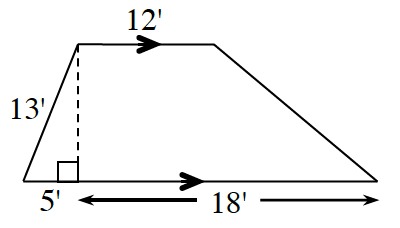Home > CCG > Chapter 2 > Lesson 2.3.2 > Problem2-115

2-115.

Find the area of the trapezoid below. What strategies did you use? Homework Help ✎Look at the Math Notes box in Lesson 2.2.4 for help.

$5^2 + c^2 = 13^2\\-25\ \ \ \ -25$

$\text{Area of a trapezoid }=\frac{1}{2}(b_{1}+b{_{2}})h$